# SAT II Math II : Law of Sines

## Example Questions

### Example Question #1 : Law Of Sines

Find the measure of angle.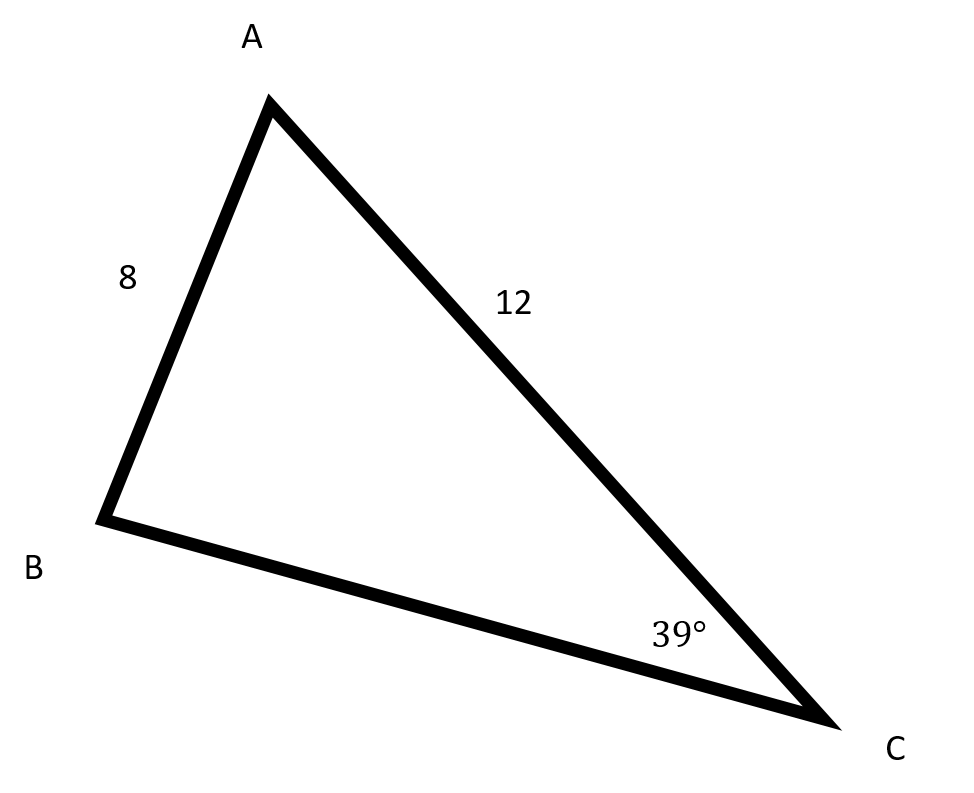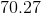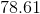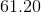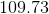Explanation:Start by using the Law of Sines to find the measure of angle.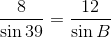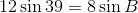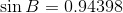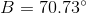Since the angles of a triangle must add up to,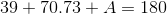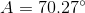### Example Question #2 : Law Of Sines

In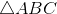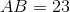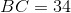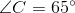Evaluate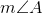(nearest degree)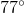Cannot be determined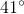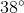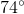Cannot be determined

Explanation:

By the Law of Sines, ifandare the lengths of two sides of a triangle, and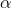and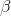the measures of their respective opposite angles,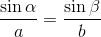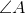and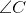are opposite sides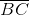and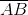, so, setting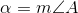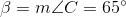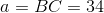, and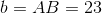: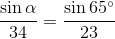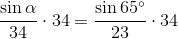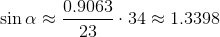However, the range of the sine function is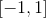, so there is no value offor which this is true. Therefore, this triangle cannot exist.